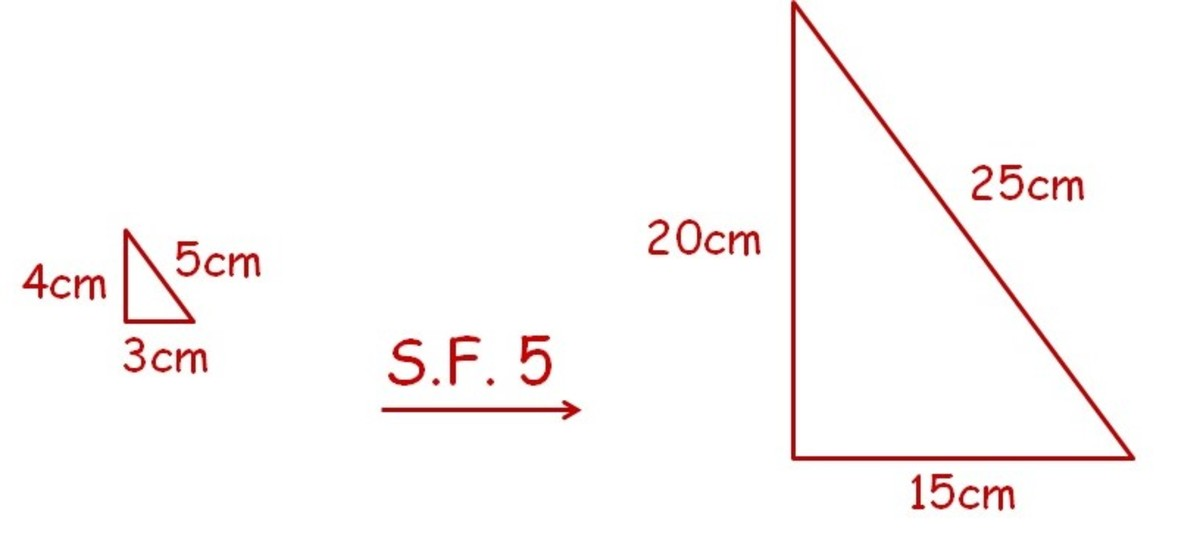# How Do Scale Factors Work for Area and Volume?

• Author:
• Updated date:

I am a former maths teacher and owner of DoingMaths. I love writing about maths, its applications, and fun mathematical facts.

## What is a Scale Factor?

When enlarging a shape or image, we use a scale factor to tell us how many times bigger we want each line/side to become. For example, if we enlarged a rectangle by scale factor 2, each side would become twice as long. If we enlarged by a scale factor of 10, each side would become 10 times as long.

The same idea works with fractional scale factors. A scale factor of 1/2 would make every side 1/2 as big (this is still called an enlargement, even though we have ended up with a smaller shape).

## Watch How to use Scale Factors with Area and Volume on the DoingMaths YouTube channelIn this picture, the shape has been scaled by a factor of 5

## Enlarging With a Scale Factor of 5

In the diagram above, the left-hand triangle has been enlarged by a scale factor of 5 to produce the triangle on the right. As you can see, each of the three side lengths of the original triangle has been multiplied by 5 to produce the side lengths of the new triangle.

## Scale Factors With Area

But what effect does enlarging by a scale factor have on the area of a shape? Is the area also multiplied by the scale factor?

Let's look at an example.

## Enlarging an Area by a Scale Factor

In the diagram above, we have started with a rectangle of 3cm by 5cm and then enlarged this by a scale factor of 2 to get a new rectangle of 6cm by 10cm (each side has been multiplied by 2).

Look at what has happened to the areas:

Original area = 3 x 5 = 15cm2

New area = 6 x 10 = 60cm2

The new area is 4 times the size of the old area. By looking at the numbers we can see why this has happened.

The length and the height of the rectangle have both been multiplied by 2, hence when we find the area of the new rectangle we now have two lots of x2 in there, hence the area has been multiplied by 2 twice, the equivalent of multiplying by 4.

Scroll to Continue

More formally, we can think of it like this:

After an enlargement of scale factor n:

New area = n x original length x n x original height

= n x n x original length x original height

= n2 x original area.

So to find the new area of an enlarged shape, you multiply the old area by the square of the scale factor.

This is true for all 2-d shapes, not just rectangles. The reasoning is the same; area is always two dimensions multiplied together. These dimensions are both being multiplied by the same scale factor, hence the area is multiplied by the scale factor squared.

## Enlarging a Volume by a Scale Factor

What about if we enlarge a volume by a scale factor?

Look at the diagram above. We have enlarged the left-hand cuboid by a scale factor of 3 to produce the cuboid on the right. You can see that each side has been multiplied by 3.

The volume of a cuboid is height x width x length, so:

Original volume = 2 x 3 x 6 = 36cm3

New volume = 9 x 6 x 18 = 972cm3

By using division we can quickly see that the new volume is actually 27 times larger than the original volume. But why is this?

When enlarging the area we needed to take into account how two multiplied sides were both being multiplied by the scale factor, hence we ended up using the square of the scale factor to find the new area.

For volume it is a very similar idea, however this time we have three dimensions to take into consideration. Again, each of these is being multiplied by the scale factor, so we need to multiply our original volume by the scale factor cubed.

More formally, we can think of it like this:

After an enlargement of scale factor n:

New volume = n x original length x n x original height x n x original width

= n x n x n x original length x original height x original width

= n3 x original volume.

So to find the new volume of an enlarged 3d shape, you multiply the old volume by the cube of the scale factor.

## Summary

In summary, the rules of enlarging areas and volumes are very easy to remember, especially if you remember how we worked them out.

If you are enlarging by a scale factor n:

Enlarged length = n x original length

Enlarged area = n2 x original area

Enlarged volume = n3 x original volume.